## Thursday, 2 March 2006

Hexadecimals are very interesting, and if you've read this post, you'll realize that they are hard to explain as well. So I decided to make another post to help in converting normal numbers to hexadecimal (okay, okay, it was an assignment as well...) Hopefully all will understand how to do this.

If take a look at my assignment that I'm supposed to do, you'll realize that there are numbers that only go up to 150, so I won't be including a '256' column in my imaginary table (the table is something like the one below, which might help in explaining what I'm trying to say).

Okay so let's start converting:
1. The first number is 12, and as you might know already if you've read through my previous post, you probably already know that there isn't a number for 12, it's a letter... So if you look at the chart on that page you are going to see that 12 is C. So the answer is C!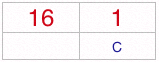2. The second number is 20. Since we can't get 20 by one number we would have to use both the 16 and the 1 place values. You can look at the chart below for your reference.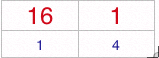Since 16x1 + 1x4 is 20 the answer is 14!
3. The third number is 60.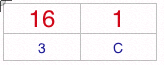Since 16x3 + 1xC(C has the value of 12) is 60 the answer is 3C!
4. The fourth number is 32.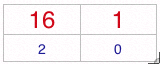Since 16x2 + 1x0 is 32 the answer is 20!
5. The fifth number is 100.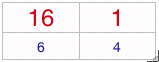Since 16x6 + 1x4 is 100 the answer is 64!
6. The sixth number is 150.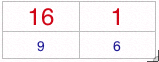Since 16x9 + 1x6 is 150 the answer is 96!
7. The seventh number is 17.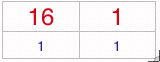Since 16x1 + 1x1 is 17 the answer is 11!

If you've paid attention to the red letters and bold words you should have no problem in grasping this concept!

Got a question, tip or comment? Send them to beyondteck+question@gmail.com and we'll try to answer it in a blog post!